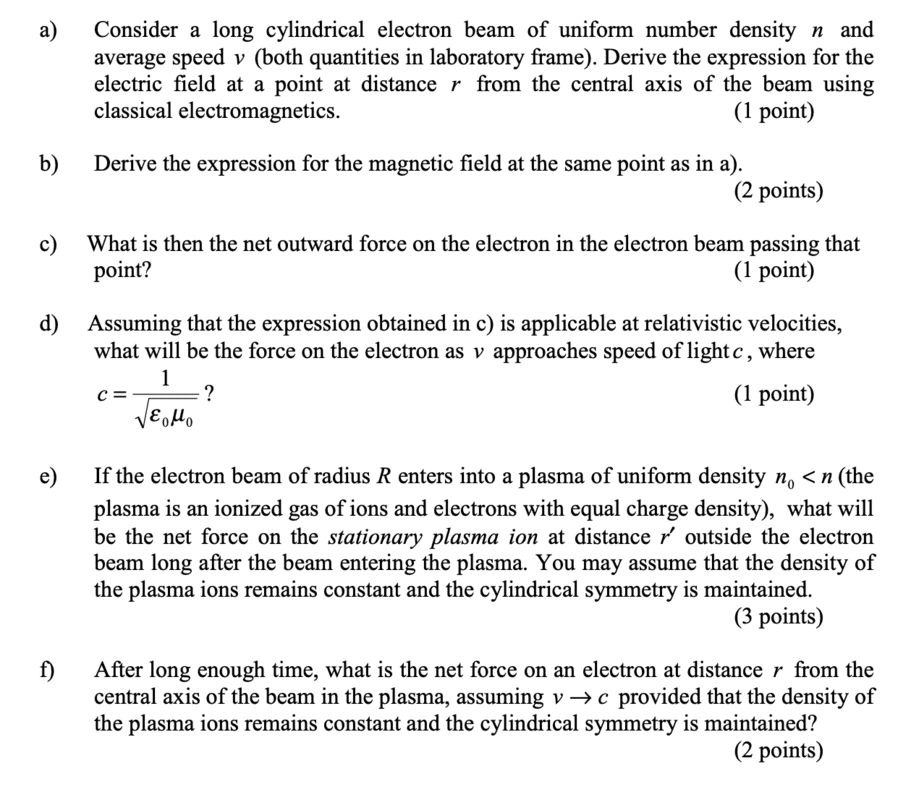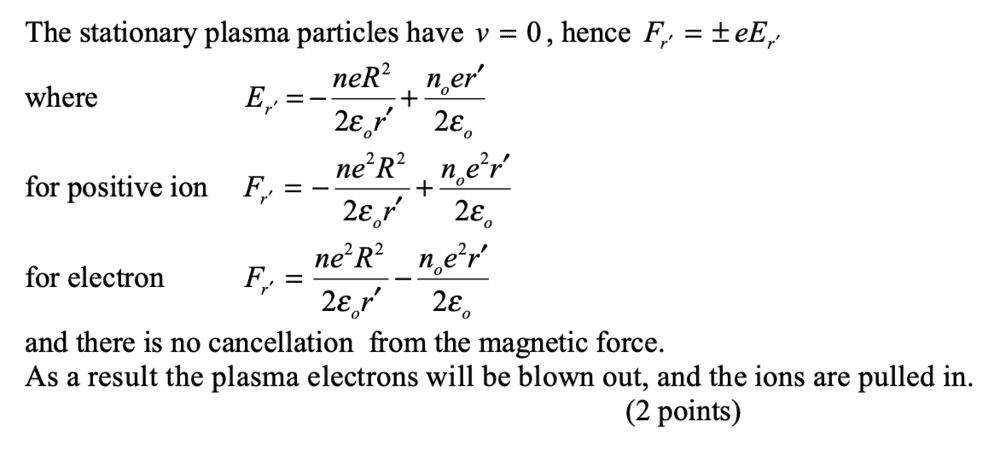# Gauss's Law and Ampere's Law -- Force on an ion in a plasma due to a nearby electron beam

phantomvommand
Homework Statement:
Please refer to the picture below
Relevant Equations:
Gauss's Law
Ampere's Law
I am only asking about part e. If you are short on time, you can read through parts a - d, to get an idea of what is happening, and then attempt part e directly.I have solved parts a - d. If you would like to check your answers, the answer to part c is [rne^2 / 2e0] [ 1 - (v/c)^2], and the answer to part d is 0.
I do not understand the following solution to part e. The term on the left represents the E-field due to the electron beam, which I understand. I suppose the term on the right is the E-field due to the positively charged ions in the plasma. However, isn't the effect of the positively charged ions in the plasma negated by the effect of the negatively charged electrons in the plasma? Thus, the term on the right should not exist. What is wrong?Homework Helper
Gold Member
2022 Award
Here’s a guess…

I suspect the key phrase in part e) is “… long after the beam entering the plasma.”

In the long-term (when a steady-state has been reached), the plasma-electrons will have been largely expelled from the region radius r’ due to repulsion by the electron beam. So what remains of the plasma are the positive ions only. The electric field at r’ is then the sum of the fields from the electron beam and positive plasma ions.

It’s not entirely consistent with the wording of question and solution, but maybe these are simply poorly written.

phantomvommand
Here’s a guess…

I suspect the key phrase in part e) is “… long after the beam entering the plasma.”

In the long-term (when a steady-state has been reached), the plasma-electrons will have been largely expelled from the region radius r’ due to repulsion by the electron beam. So what remains of the plasma are the positive ions only. The electric field at r’ is then the sum of the fields from the electron beam and positive plasma ions.

It’s not entirely consistent with the wording of question and solution, but maybe these are simply poorly written.

Thanks for the reply. May I know how the electrons can be expelled, given that the positive ions also exert an attractive force on them?

Homework Helper
Gold Member
2022 Award
Thanks for the reply. May I know how the electrons can be expelled, given that the positive ions also exert an attractive force on them?
Suppose you put a negative charge, -q, inside a hollow metal spherical shell. Some of the metal’s electrons are pushed out (‘expelled’) onto the outer surface, giving an outer-surface charge of -q and leaving an inner-surface charge of +q.

Not the best analogy, but the underlying principle is the same as the electron-beam and plasma in your question.

The ‘expulsion’ of plasma-electrons is from the region around the electron-beam into more distant regions.